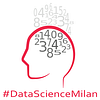# TensorFlow Dev Summit 2019 Summary and Deep Diving into GANs

Source: Deep Learning on Medium

“TensorFlow 2.0: usability and portability”

On 4th June 2019 at Google Italy, Data Science Milan has gathered its community to view together some talks of TensorFlow Dev Summit 2019 and to explore new features applied into GANs.

“TensorFlow Dev Summit 2019”

TensorFlow afford you to build everything but sometimes TensorFlow 1.0 is a little bit hard to use, for example applying a “session.run()”, is not the most natural thing to do if you coming from the Python programming world.

TensorFlow 2.0 is focused on usability: easy model building with Keras and eager execution, robust model deployment in production and compatibility throughout the TensorFlow ecosystem, simplifying API and reduction of duplication.

Keras is been deeply integrated and extended in TensorFlow, you can use all of the advanced features in Tensorflow directly from “tf.keras”. Many APIs have been consolidated across TensorFlow, under the Keras heading reducing duplicative classes and making easier to know what you should use and when. TensorFlow 2.0 is innovative in eager execution, because with TensorFlow 1.0 you need to create a computational graph and run it calling “session.run()”, TensorFlow 2.0 executes it eagerly (like Python normally does) so there is a transition from a graph-based approach into an object oriented approach.

One of the biggest innovation is the changing of the programming model with which you build graphs in TensorFlow. That model where you first add a bunch of nodes to a graph and then rely on “session.run()” to prune things out of the graph, to figure out the precise things you want to run, are replaced with a simpler model based on the notion of a function. With TensorFlow 2.0 you can create a composition of Python operations, call it using “tf.function()” and it runs as a single graph. Benefits of this graph mode are in performance and portability. Look at the complete playlist.

“Deep Diving into GANs: from theory to production”, by Paolo Galeone, Google Developer Expert in Machine Learning

Have a look at GAN: Theory and Applications Notebook to understand how GANs work and also you can take a peek both:

GANs are based on Game theory where two agents are competing each other: a generator creates random samples to map a data distribution and a discriminator is a classifier that discriminates between samples from the training set and free samples created by the generator.

The architecture consists on two neural network trained simultaneosly: given a noise input for the generator, the dicriminator compares the output from the generator with true data and gives a feedback used to update parameters of both networks, so GANs can be viewed as one network.

To notice that generator learns without real data as in a reinforcerment learning process.

There are two cost functions, one for the discriminator and another one for the generator. The goal of the discriminator is to maximize two terms: in the first one the probability to classify in the right way samples coming from the real data distribution, in the second term the probability to classify fake samples generated by the generator.

The generator aim to fool the discriminator creating true data like the real one, maximizing the probability to generate better samples as possible, so playing the same game but in a opposite direction.

To reach the Nash equilibrium and to make the training process more stable are used minibatch stochastic gradient descent. These type of GANs are known as Unconditional GANs with the following limit: no control on modes of the data being generated.

To overcome this lack there are Conditional GANs built using a label that allows the generator to create a fake sample with a specific condition or characteristics (label) rather than a generic sample from unknown noise distribution.

The training process can be considered ended when the discriminator is completely fooled by the generator.

After the theory introduction, Paolo has explained how to build GANs from scratch using TensorFlow 2.0 API. Look at the Writing a GAN from scratch Notebook.

The goal is to learn a certain data distribution: a Gaussian Distribution with mean equal to zero and a standard deviation equal to 0.1.

Generator and Discriminator are built with a completely arbitrary architecture and a linear activation for the output, as mandatory. These models are developed with Keras functional API, using Keras layers as functions. Look at the video.

Written by Claudio G. Giancaterino